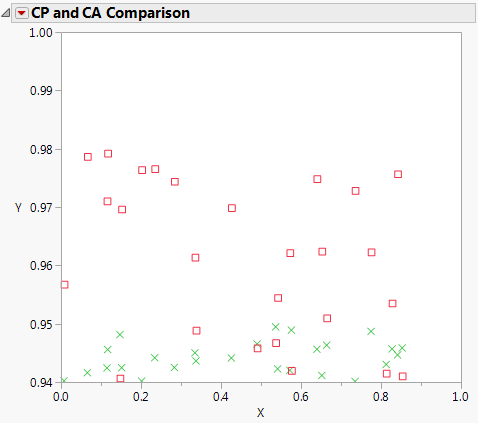Scripting Guide > Data Tables > Rows > Row States
Publication date: 05/24/2021

## Row States

There is a special data element type called a row state that stores various attributes in the data table. Row states can indicate the following:

Whether a row is selected, excluded, hidden, or labeled

Which marker type, color, shade, and hue to use for graphs

In JSL, you can use row state functions to manipulate row states.

Row states change how JMP works with your data. Table 9.5 explains each row state. Remember that you can use several row states at once to get the combination of effects that you want.

Table 9.5 Row States

Row states

How they affect results

Excluded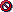If rows are excluded, JMP omits them from calculations for statistical analyses (text reports and charts). Results are the same as if the data was not entered. However, points are still included in plots. (To omit points from plots, use Hide. To omit points from all results, use both Exclude and Hide.)

Hidden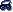If rows are hidden, JMP does not show them in plots. However, the rows are still included in text reports and charts. (To omit points from reports and charts, use Exclude. To omit points from all results, use both Exclude and Hide.)

Labeled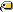If rows are labeled, JMP places row number labels, or the values from a designated Label column, on points in scatterplots.

Color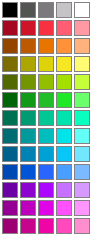If rows have colors, JMP uses those colors to distinguish the points in scatterplots.

Marker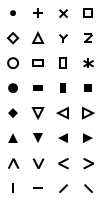If rows have markers, JMP uses those markers to distinguish the points in scatterplots.

Selected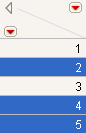If rows are selected, JMP highlights the corresponding points and bars in plots and charts.

Note: For row state messages, omitting the argument toggles the option: if the option is off, the message turns it on, and if the option is on, the message turns it off. You can also use the "toggle", "switch", or "flip" argument, as in r << Exclude( "toggle" ).

When you give a row state function a number as its argument (or an expression that evaluates to a number), the function interprets that number as an index to its possible values.

Table 9.6 shows a comparison chart of the different row state functions, so that you can see which functions convert row states to numbers and numbers to row states. It also includes the numbers that you can use with each function.

Table 9.6 Row State Functions

Numbers

Convert from numbers to row states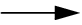Row states

Convert from row states to numbersNumbers

1 or 0

Excluded State(n)

ExcludedExcluded(rowstate)

1 or 0

1 or 0

Hidden State(n)

HiddenHidden(rowstate)

1 or 0

1 or 0

Labeled State(n)

LabeledLabeled(rowstate)

1 or 0

1 or 0

Selected State(n)

SelectedSelected(rowstate)

1 or 0

0 to 31

Marker State(n)

MarkerMarker Of(rowstate)

0 to 31

0 to 84

(0–15 basics, 16–31 dark, 32–47 light, 48–63 very dark, 64–79 very light, 80–84 grays)

Color State(n)

ColorColor Of(rowstate)

0 to 84

(0–15 basics, 16–31 dark, 32–47 light, 48–63 very dark, 64–79 very light, 80–84 grays)

0–11

(rainbow order)

Hue State(n)

Hue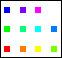–2 to 2

(dark to light)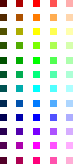### Assign Row States

To assign a row state using JSL, use Select Where to indicate which rows are affected, and then specify which row state to assign to the rows. In the following example, marker type 5 (a triangle) is assigned to rows in which sex is “F”.

`dt = Open( "\$SAMPLE_DATA/Big Class.jmp" );`
`dt << Select Where( :sex == "F" ) << Markers( 5 );`

You can also use a formula to assign row states. Here is how you would do it in the data table:

1. Create a row state column.

2. Add a formula to the column that assigns a marker to each row in which sex is “F”.

3. In the Column panel, right-click the star next to the row state column and select Copy to Row States.

The JSL equivalent would be something like this:

`dt = Open( "\$SAMPLE_DATA/Big Class.jmp" );`
`dt << New Column( "Row State",`
`	Row State,`
`	Set Formula( If( :sex == "F", Marker State( 4 ) ) )`
`);`
`Column( "Row State" ) << Copy To Row States();`

### Store Row State Information

A row state column is a dedicated column that stores row state information, but does not put the information into effect. You can then use For Each Row to put the row state column into effect. For more information about row state columns, see Store Information in Row State Columns in Using JMP.

The following example creates a row state column then puts the row states into effect:

1. Submit this line to start a new data table:

`dt = New Table( "Row State Testing" );`

2. Submit these lines to add row states to a column and add ten rows:

`dt << New Column( "Row State Data", Row State, Set Formula( Color State( Row() ) ) );`
`dt << Add Rows( 10 );`

3. Submit this line to put the row states into effect:

`For Each Row( Row State() = :Row State Data );`

Figure 9.17 Table with No Row States (left) and Table with Row States (right)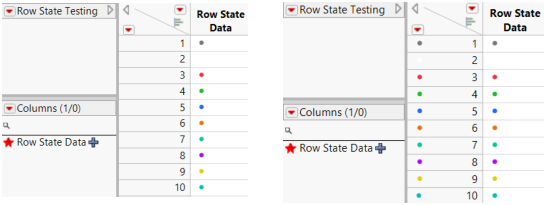This action replaces any row states in effect with the row state combination from the row state column. For more information about how to change selected attributes of a row state without changing or canceling others, see Set One Characteristic and Cancel Others.

### Set or Get Row States

From JSL, you can set or get row states directly using the Row State function. You can set or get the state for row n with Row State(n). If you do not supply an argument, you set or get the current row. To work with all rows, either use a For Each Row loop or work with formula columns.

To set a row state, place the row state expression on the left of an assignment (L-value):

`Row State( 1 ) = Color State( 3 ); // make row 1 red`
`Row() = 8; Row State() = Color State( 3 ); // make the 8th row red`
`For Each Row( Row State() = Color State( 3 ) ); // make each row red`

To specify the data table, include a data table reference as the first argument:

`dt1 = Open( "\$SAMPLE_DATA/Big Class.jmp" );`
`dt2 = Open( "\$SAMPLE_DATA/San Francisco Crime.jmp" );`
`Row State( dt1, 1 ) = Color State( 3 ); // make row 1 red;`

To get a row state, place the row state expression on the right side of an assignment:

`x = Row State( 5 );  // put the row state of row 5 into x`
`x = Row State();   // row state of current row`

#### Notes on Setting Row States

Be careful whether you set every aspect of Row State() or just one aspect of it, such as Color Of( Row State() ). To see how this works, first color and mark all the rows:

`dt1 = Open( "\$SAMPLE_DATA/Big Class.jmp" );`
`For Each Row(`
`	Row State() = Combine States( Color State( Row() ), Marker State( Row() ) ) );`

And now observe the difference between setting one attribute of a row state:

`Color Of( Row State( 1 ) ) = 3; // make row 1 red without changing marker`

And setting every aspect of a row state to a single state:

`Row State( 1 ) = Color State( 5 ); // make row 1 blue and remove its marker`

To copy all the current row states into a row state column:

`dt1 = Open( "\$SAMPLE_DATA/Big Class.jmp" );`
`For Each Row(`
`       dt1,`
`       Row State() = Combine States(`
`              Color State( Row() ),`
`              Marker State( Row() )`
`       )`
`);`
`dt1 << New Column( "rscol", "Row State", Set Formula( Row State() ) );`

To copy several but not all of the current row states into a row state column, use a script like the following (commenting out or omitting any states that you do not want):

`New Column( "rscol2",`
`	Set Formula(`
`		Combine States(`
`			Color State( Color Of() ),`
`			Excluded State( Excluded() ),`
`			Hidden State( Hidden() ),`
`			Labeled State( Labeled() ),`
`			Marker State( Marker Of() ),`
`			Selected State( Selected() )`
`		)`
`	)`
`);`

To set a component of a row state:

`Color Of( Row State( i ) ) = 3; // change color to red for row i`
`Selected( Row State( i ) ) = 1; // select the ith row state and set to 1`

You can see from the example above that some of the functions convert numbers into states, and others convert states into numbers. Here are some helpful hints for remembering which are which:

Number-to-state functions have the word “State”

Functions that take number arguments and either return states or accept state assignments all have the word “State” in their names: Row State, As Row State, Color State, Combine States, Excluded State, Hidden State, Hue State, Labeled State, Marker State, Selected State, Shade State.

State-to-number functions are one word or have the word “Of”

Functions that take row state arguments (and assume that the argument Row State() if none is given) and functions that return or are set to numbers are either one word, or their second word is “Of”: Color Of, Excluded, Hidden, Labeled, Marker Of, Selected.

Table 9.6 is a helpful comparison chart for these functions.

The following lines are equivalent to their interactive commands:

`Copy From Row States`
`Add From Row States`
`Copy To Row States`
`Add To Row States`

#### Examples of Getting Row States

For example, create the following table containing row states:

`dt = Open( "\$SAMPLE_DATA/Big Class.jmp" );`
`dt << New Column( "Row State Data", Row State, Set Formula( Color State( Row() ) ) );`
`dt << Add Rows( 10 );`
`For Each Row( Row State() = :Row State Data );`

To get row states, submit the following lines:

`Row State( 1 ); // returns row state for row 1`
`Row() = 8; Row State();  // returns row state for current (8th) row`
`For Each Row( Print( Row State()) ); // returns the row states for each row`

To get a row state and store it in a global, place Row State() on the right side of an assignment. Add the following lines to the preceding script:

`::x = Row State( 1 ); // put the row state of row 1 in x, a global`
`Row() = 8; ::x = Row State();  // put the row state of the 8th row in x`
`Show( x ); // returns x = Color State( 8 )`
`dt << New Column( "rscol", Row State) ;`

// put row states in rscol (a row state column)

`For Each Row( :rscol = Row State() );`

To get a component of a row state, place the row state expression on the right side of an assignment and also use one of the L-value operators:

`x = Selected( Row State() ); // selection index of current row selected`

#### Get and Set Multiple Characteristics at Once

You can get or set many characteristics at once by combining state settings inside Combine States(). You can also get or set each characteristic one at a time, the ultimate row state being the accumulation of characteristics. The following example sets green Y markers for males, but hides them in plots for now. It also sets red X markers for females but shows them in plots.

`dt = Open( "\$SAMPLE_DATA/Big Class.jmp" );`
`For Each Row(`
`	If( sex == "M",`
`		/* then */ Row State() = Combine States(`
`			Color State( 4 ), Marker State( 6 ), Hidden State( 1 ) ),`
`		/* else */ Row State() = Combine States(`
`			Color State( 3 ), Marker State( 2 ), Hidden State( 0 ) ) ) );`

Get the row state for one row, such as the 6th:

`Row State( 6 );`

Combine States(Hidden State(1), Color State(4), Marker State(6))

Notice that JMP returns a Combine State() combination. This is because a row state datum is not just the state of one characteristic, such as color, but the cumulative state   of all the characteristics that have been set: exclusion, hiding, labeling, selection, markers, colors, hues, and shades. A list of such characteristics is called a row state combination.

Just as there can be many row state characteristics in effect, a row state column can have multiple characteristic row states as its values.

#### Set One Characteristic and Cancel Others

In addition to the overall Row State() function for getting or setting all the characteristics of a row state, there are separate functions to get or set one characteristic at a time preemptively. That is, to give a row one characteristic, canceling any other characteristics that might be in effect. The functions that set one characteristic and cancel others are Color State, Combine States, Excluded State, Hidden State, Hue State, Labeled State, Marker State, Selected State, Shade State.

For example, to make row 4 be hidden only:

`Row State( 4 ) = Hidden State( 1 );`

#### Set or Get One Characteristic at a Time

A row state is not just one characteristic, but many. To work with just one characteristic at a time, use one of the L-value operators with Row State on either side of the equal sign. The side of the equal sign depends on whether you want to get or set a characteristic. There is an L-value operator for each of the following characteristics: Color Of, Excluded, Hidden, Labeled, Marker Of, Selected.

This example hides row 4 without affecting any other characteristics:

`Hidden( Row State( 4 ) ) = 1`

This example stores the color of row 3 without getting any other characteristics:

`::color = Color Of( Row State( 3 ) );`

### Identify Row State Changes

The MakeRowStateHandler message (sent to a data table object) obtains a callback when the row states change. For example,

`f = Function( {X}, Show( x ) );`
`obj = dt << Make Row State Handler( f );`

Then when you select a group of rows, the row numbers of any row whose row state changed are sent to the log. For example:

x:[3, 4, 28, 40, 41]

When a group is highlighted, it might call the handler twice, once for rows whose selection is cleared, then again for the new selection.

### Exclude, Hide, Label, and Select

This section discusses conditions that have Boolean states (either on or off). These conditions include: excluding, hiding, labeling, and selecting rows.

Excluded gets or sets an excluded index. The index is 1 for true or 0 for false, indicating whether each row is excluded.

Hidden gets or sets a hidden index, which is 1 for hidden or 0 for not hidden.

Labeled gets or sets a labeled index, which is 1 for labeled or 0 for not labeled.

Selected gets or sets a selected index, which is 1 for selected or 0 for not selected.

The following examples illustrate these conditions:

`Excluded( Row State() ); // returns 1 if current row is excluded, 0 if not`
`Hidden(); 									// returns 1 if current row is hidden, 0 if not`
`Labeled( Row State() );  // returns 1 if current row is labeled, 0 if not`
`Selected();            									// returns 1 if current row is selected, 0 if not`
` `
`Excluded( Row State() ) = 1; // exclude current row`
`Hidden() = 0;             										 // unhide current row`
`Labeled( Row State() ) = 1;  // label current row`
`Selected() = 0;            										// deselect current row`

Remember that these functions assume the argument Row State() if none is given.

Excluded State, Hidden State, Labeled State, and Selected State do the reverse; they get or set a row state condition as true or false according to the argument. Nonzero values set the row state to true, and zero values set it to false. Missing values result in no change of state.

`Row State() = Excluded State( 1 ); // change current row state to excluded`
`Row State() = Hidden State( 0 );   // change current row state to not hidden`
`Row State() = Labeled State( 1 );  // change current row state to labeled`
`Row State() = Selected State( 0 );`
`// change current row state to not selected`

Notice that the first two expressions above replace the row state with just the exclusion or just the unhiding, so that any preexisting row state characteristics are lost. More commonly, you would issue the state commands for all of the characteristics that you want inside a Combine States. The following example changes the third row to hidden and a green square marker:

`dt = Open( "\$SAMPLE_DATA/Big Class.jmp" );`
`Row State( 3 ) = Combine States(`
`	Color State( 4 ),`
`	Marker State( 3 ),`
`	Hidden State( 1 ) );`

Another common way to use a -State command would be in a row state data column whose values could be added to the row state (for cumulative characteristics). The following example excludes each odd numbered row:

`dt = Open( "\$SAMPLE_DATA/Big Class.jmp" );`
`dt << New Column( "myExcl",`
`	Row State,`
`	Set Formula( Excluded State( Modulo( Row(), 2 ) ) )`
`);`
`For Each Row( Row State() = Combine States( :myExcl, Row State() ) );`

### Clear Row States

Clear Row States() removes row states from the data table. The following script assigns a row state to each row and then removes the row state.

`dt = Open( "\$SAMPLE_DATA/Big Class.jmp" );`
`For Each Row( Row State() = Color State( Row() ) );`
`Wait( 3 ); // pause for demonstration purposes`
`dt << Clear Row States();`

To clear row states for selected rows, select the rows first and then clear the selected row states.

`dt = Open( "\$SAMPLE_DATA/Big Class.jmp" );`
`For Each Row( Row State() = Color State( Row() ) );`
`dt << Select Rows( [ 5 ] );`
`Wait( 3 ); // pause for demonstration purposes`
`dt << Clear Selected Row States();`

### Colors and Markers

This section discusses conditions that have many choices. These conditions include: coloring, adding markers, different hues, or different shades to rows.

Color Of() returns or sets the color index. The color index is a number from the JMP color map that corresponds to the row state, or a missing value if there is no assigned color.

`Color Of( Row State() );   // returns color index for current row`
`Color Of() = 4;           // set current row to Color 4`

Similarly, Marker Of returns or sets the marker index. The marker index is a number from the JMP marker map that corresponds to the active marker, or a missing value if there is no assigned marker.

`Marker Of();             											// returns marker index for current row`
`Marker Of( Row State() ) = 4; // set current row to Marker 4`

Both Color Of() and Marker Of() accept any row state expression or column or Row State() as arguments. They also assume the argument Row State() if none is given (some examples are shown with, and some without).

Color State() and Marker State() are similar to Color Of() and Marker Of(), except they work in the opposite direction. Where the -Of functions turn actual states into indices, the -State functions turn indices into states.

`Row State() = Color State( 4 );  // change current row to green`
`Row State() = Marker State( 4 ); // change current row to the diamond marker`

Notice that the last two commands replace the row state with just the color or just the marker, so that any preexisting row state characteristics are lost. More commonly you would issue the -State commands for all the characteristics that you want inside a Combine States(). The following example puts a green square marker in the third row and hides the row:

`dt = Open( "\$SAMPLE_DATA/Big Class.jmp" );`
`Row State( 3 ) = Combine States(`
`	Color State( 4 ),`
`	Marker State( 3 ),`
`	Hidden State( 1 ) );`

The following script shows the standard JMP markers, which are numbered 0–31. Indices outside the range 0–31 have undefined behavior.

`New Window( "Markers",`
`	Graph Box(`
`		FrameSize( 300, 300 ),`
`		Y Scale( -1, 16 ),`
`		X Scale( 0, 7 ),`
`		For(`
`			i = 0;`
`			jj = 15;,`
`			i < 16;`
`			jj >= 0;,`
`			i++;`
`			jj--;, // 16 rows, 2 columns`
`			Marker Size( 3 );`
`			Marker( i, {1, jj + .2} ); // markers 0-15`
`			Marker( i + 16, {4, jj + .2} ); // markers 16-31`
`			Text( {1.5, jj}, "marker ", i ); // marker labels 0-15`
`			Text( {4.5, jj}, "marker ", i + 16 ); // marker labels 16-31`
`		)`
`	)`
`);`

Figure 9.18 JMP Markers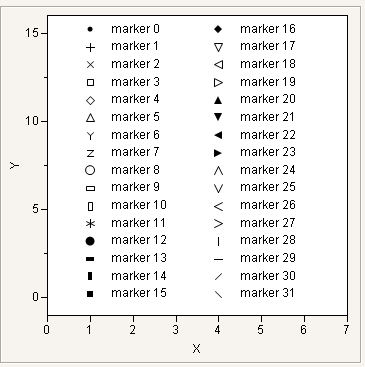JMP colors are numbered 0 through 84.

The first 16 named colors are the basic colors. See the script below.

Numbers higher than 16 are darker or lighter shades of the basic colors.

Indices outside the range 0–84 have undefined behavior.

The following script illustrates the standard JMP colors:

`Text Color( 0 );`
`New Window( "Colors",`
`	Graph Box(`
`		FrameSize( 640, 400 ),`
`		Y Scale( -1, 17 ),`
`		X Scale( -3, 12 ),`
`		k = 0;`
`		For( jj = 1, jj <= 12, jj += 2,`
`			l = 15;`
`			For( i = 0, i <= 15 & k < 85, i++,`
`				thiscolor = Color To RGB( k );`
`				Fill Color( k );`
`				thisfill = 1;`
`				If( thiscolor == {1, 1, 1},`
`					Pen Color( 0 );`
`					thisfill = 0;`
`				,`
`					Pen Color( k )`
`				);`
`				Rect( jj, l + .5, jj + .5, l, thisfill );`
`				Text( {jj - 1, l}, "color ", k );`
`				k++;`
`				l--;`
`			);`
`		);`
`		jj = -2;`
`		color = {"Black", "Gray", "White", "Red", "Green", "Blue", "Orange", "BlueGreen",`
`		"Purple", "Yellow", "Cyan", "Magenta", "YellowGreen", "BlueCyan", "Fuschia", "Black"};`
`		For(`
`			i = 0;`
`			l = 15;, i <= 15 & l >= 0,`
`			i++;`
`			l--;,`
`			Text( {jj, l}, color[i + 1] )`
`		);`
`	) );`

Figure 9.19 JMP ColorsIf you prefer to use RGB values, each color should be a list with percentages for each color in red, green, blue order. For example, the following percentages produce a teal color:

`Pen Color( {.38,.84,.67} ); // a lovely teal`

Hue State and Shade State together are an alternative to Color State for choosing colors. You cannot select black, white, or the shades of gray when you use Hue State. For these, you must use Shade State alone, or Color State.

The following script demonstrates how hue and shade values relate to colors:

`New Window( "Hues and Shades",`
`	Graph Box(`
`		FrameSize( 600, 300 ),`
`		Y Scale( -3, 3 ),`
`		X Scale( -2, 12 ),`
`		k = 0;`
`		For( h = 0, h < 12, h++,`
`			For( s = -2, s < 3, s++,`
`				myMk = Combine States( Hue State( h ), Shade State( s ), Marker State( 15 ) );`
`				Marker Size( 3 );`
`				Marker( myMk, {h, s} );`
`			)`
`		);`
`		Text( Center Justified, {5, 2.5}, " <--- Hues 0-11 ---> " );`
`		Text( Right Justified,`
`			{-.5, -2}, "Shade -2", {-.5, -2.25}, "(Very dark)",`
`			{-.5, -1}, "Shade -1", {-.5, -1.25}, "(Dark)",`
`			{-.5, 0}, "Shade 0", {-.5, -.25}, "(Basic hue)",`
`			{-.5, 1}, "Shade 1", {-.5, .75}, "(Light)",`
`			{-.5, 2}, "Shade 2", {-.5, 1.75}, "(Very light)"`
`		);`
`	)`
`);`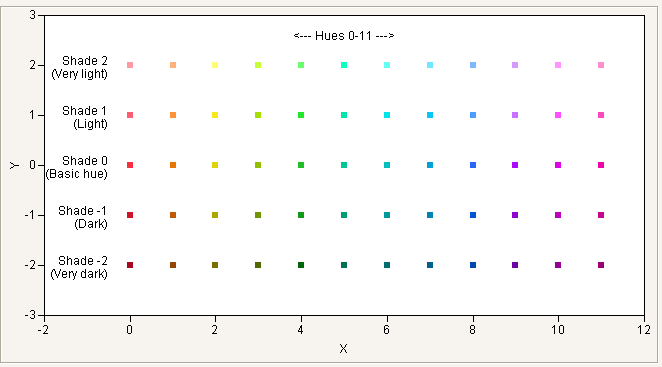There are no -Of functions for Hue and Shade. Color Of returns the equivalent Color State index for a color row state that has been set with Hue State or Shade State. For example, the following example gives rows 4 and 5 the same dark red marker:

`Row State( 4 ) = Combine States( Hue State( 0 ), Shade State( -1 ), Marker State( 12 ) );`
`Row State( 5 ) = Combine States(`
`	Color State( Color Of( Row State( 4 ) ) ),`
`	Marker State( Marker Of( Row State( 4 ) ) )`
`);`

#### Row State and Matrices Example

In the following example, row state values are prepared ahead and passed to the Marker routine, along with matrices of coordinates.

`dt = New Table( "Artificial CP and CA data",`
`	Add Rows( 26 ),`
`	New Column( "cover_cp",`
`		Numeric,`
`		"Continuous",`
`		Formula( Random Uniform() / 100 + 0.94 )`
`	),`
`	New Column( "cover_ca",`
`		Numeric,`
`		"Continuous",`
`		Formula( Random Uniform() * 0.04 + 0.94 )`
`	),`
`	New Column( "p", Numeric, "Continuous", Formula( Random Uniform() ) )`
`);`
`dt << Run Formulas;`
`greenMark = Combine States( Marker State( 2 ), Color State( 4 ) );`
`redDiamond = Combine States( Marker State( 3 ), Color State( 3 ) );`
`New Window( "CP and CA Comparisons",`
`	Graph Box(`
`		Title( "CP and CA Comparison" ),`
`		FrameSize( 400, 350 ),`
`		X Scale( 0, 1 ),`
`		Y Scale( 0.94, 1 ),`
`		For Each Row(`
`			Marker( greenMark, {p, cover_cp} );`
`			Marker( redDiamond, {p, cover_ca} );`
`		)`
`	)`
`);`

Figure 9.21 Example of Row States Values and Matrices